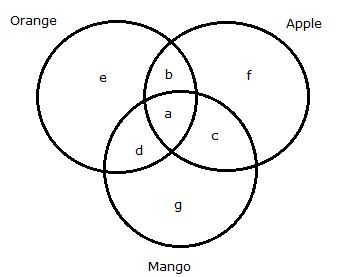# NIACL AO Prelims – Quantitative Aptitude Questions Day- 72

Dear Readers, Bank Exam Race for the Year 2019 is already started, To enrich your preparation here we have providing new series of Practice Questions on Quantitative Aptitude – Section. Candidates those who are preparing for NIACL AO Prelims 2019 Exams can practice these questions daily and make your preparation effective.

[WpProQuiz 4818]

Directions (1-5): Find the wrong number in the following series.

1) 960, 192, 48, 16, 8, 4

a) 16

b) 8

c) 4

d) 48

e) 192

2) 8640, 1440, 288, 72, 24, 6

a) 1440

b) 288

c) 72

d) 6

e) 24

3) 178, 177, 187, 196, 260, 285, 501

a) 187

b) 177

c) 196

d) 260

e) 285

4) 24, 93, 290, 551, 844, 1152

a) 1152

b) 844

c) 551

d) 290

e) 93

5) 24, 25, 52, 159, 640, 3200

a) 25

b) 52

c) 159

d) 640

e) 3200

Directions (6-10): Read the following passage carefully and answer the given questions.

There are total 1800 students in a class. Each students like one or more fruits among three fruits viz. Apple, Orange and Mango. 48% of the students like Apple. 12% of the students like Orange and Mango both but not Apple. 18% of the students like Orange and Apple both.

6) If 10% of the students like all the three fruits, find the number of students who like both Orange and Apple but not Mango.

a) 180

b) 125

c) 144

d) 169

e) 216

7) If number of students who like only Apple is 18% of the total number of students who like more than one fruit

a) 814

b) 756

c) 724

d) 658

e) 624

8) If the number of students who like only Mango is 16% of the total number of students, find the number of students who like only Orange.

a) 576

b) 448

c) 326

d) 432

e) 288

9) If 24% of the total number of students likes only Orange, find the total number of students who like Orange.

a) 972

b) 846

c) 788

d) 652

e) 576

10) Find the number of students like Apples

a) 764

b) 896

c) 694

d) 592

e) 864

Directions (1-5):

The pattern of the following series is:

960/5 = 192

192/4 = 48

48/3 = 16

16/2 = 8

8/1 = 8 (Not 4)

The pattern of the following series is:

8640/6 = 1440

1440/5 = 288

288/4 = 72

72/3 = 24

24/2 = 12 (Not 6)

The pattern of the following series is:

The pattern of the following series is:

24 * 1 + 1 =25

25 * 2 + 2 =52

52 * 3 + 3 =159

159 * 4 + 4 = 640

640 * 5 + 5 = 3205 (not 3200)

Directions (6-10):

Total students = 1800Total students = 1800

(a + b + c + d + e + f + g) = 1800 — (1)

Number of students like apple = 48/100 * 1800 = 864

(a + b + c + f) =864 —- (2)

Number of students like Orange and Mango both but not Apple = 12/100 * 1800

= 216

= > d = 216 — (3)

Number of students like Orange and Apple both = 18/100 * 1800

= 324

= > (a + b) =324 —- (4)

Substitute equation (4) in equation (2), we get

(c + f) = 864 – 324 = 540 —- (5)

Substitute the equations (3), (4) and (5) in equation (1), we get

(324 + 216 + 540 + e + g) = 1800

(e + g) = 1800 – 324 – 216 – 540

(e + g) = 720 —- (6)

Number of students like all the three fruits = 10/100 * 1800

= 180

= > a = 180

According to the question find the value of b,

Substitute a’s value in equation (4), we get

=> b = 324 – 180 = 144

Number of students like only Apple = 18/100 * 1800 = 324 = f

According to the question we need to find the value of (a + b + c + d)

Substitute the values of (e + g + f) in equation (1), we get

(a + b + c + d + e + f + g) = 1800

(a + b + c + d) = 1800 – 324 – 720

(a + b + c + d) = 756

Number of students like only Mango = 16/100 * 1800

= 288 = g

According to the question we need to find the value of e

Substitute the value of g in equation (6), we get

= > e = 720 – 288

= > e = 432

Number of students likes only Orange = 24/100 * 1800 = e

= > e = 432

According to the question we need to find the value of (a + b + d + e)

= > 324 + 216 + 432

= > 972

According to the question we need to find the value of (a + b + c + f)

= > 324 + 540

= > 864